Courses

# RD Sharma Solutions -Page No.9.21, Ratio Proportion And Unitary Method, Class 6, Maths Class 6 Notes | EduRev

## Class 6 : RD Sharma Solutions -Page No.9.21, Ratio Proportion And Unitary Method, Class 6, Maths Class 6 Notes | EduRev

The document RD Sharma Solutions -Page No.9.21, Ratio Proportion And Unitary Method, Class 6, Maths Class 6 Notes | EduRev is a part of the Class 6 Course RD Sharma Solutions for Class 6 Mathematics.
All you need of Class 6 at this link: Class 6

#### PAGE NO 9.21:

Question 11: If a : b = 2 : 5, find the value of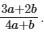a/b =  2/5  ...(1)

Now,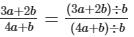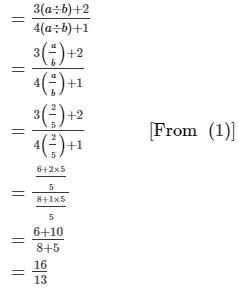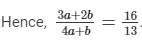Question 12: The mean proportional of a and b is 10 and the value of a is four times the value of b. If a > 0, b > 0, find the value of a + b?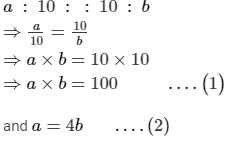From (1) and (2),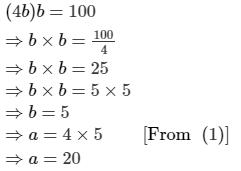∴ a+b=20+5 =25
Hence, a + b = 25.

Question 13: A given quantity of rice is sufficient for 60 persons for 3 days. How many days would the rice last for 18 persons?

ANSWER: A given quantity of rice is sufficient for 60 persons for 3 days.

A given quantity of rice is sufficient for 1 person for 3 × 60 days.

A given quantity of rice is sufficient for 18 persons for  3×60/18 = 10 days.

Hence, the rice last for 10 days for 18 persons.

Question 14: 12 men can reap a field in 25 days. In how many days can 20 men reap the same field?

ANSWER: 12 men can reap a field in 25 days.
1 man can reap a field in 25 × 12 days.
20 men can reap a field in 25×12/20=15 days.

Hence, 20 men can reap the same field in 15 days.

Question 15: Shikha and Aarushi are sisters. The ratio of their ages is 3 : 4. Their parents give them Rs. 1400 to share in the ratio of their ages. How much does each of them recieve?

ANSWER: Let the age of Shikha be 3x and the age of Aarushi be 4x.

According to the question,

3x + 4x = 1400

⇒ 7x = 1400

⇒ x=1400/7 = 200

Hence, Shikha recieves 3 × 200 = 600 rupees
and Aarushi recieves 4 × 200 = 800 rupees.

Question 16: The ratio of copper and zinc in an alloy is 5 : 3. If the weight of copper in the alloy is 30.5 g, find the weight of zinc in it.

ANSWER: Let the weight of copper be 5x and the weight of zinc be 3x.
According to the question,
5x = 30.5 g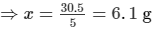Hence, the weight of zinc in the alloy is 3×6.1=18.3 g

Question 17: Divide Rs. 3450 among A, B and C in the ratio 3 : 5 : 7.

ANSWER: Let the value of A be 3x, the value of B be 5x and the value of C be 7x.

According to the question,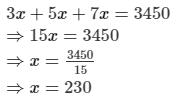Hence, the value of A is 3 × 230 = Rs.690,
the value of B is 5 × 230 = Rs.1150,
and the value of C is 7 × 230 = Rs.1610.

Question 18: 40 men can finish a piece of work in 26 days. How many men will be needed to finish it in 16 days?

ANSWER: 26 days are required to finish a piece of work by 40 men.
1 day is required to finish a piece of work by 40 × 26 men.
16 days are required to finish a piece of work by 40×26/16 = 65 men.

Hence, 65 men will be needed to finish a piece of work in 16 days.

Question 19: Tropical fruit juice is mixed from mango, papaya and orange juice in the ratio 3 : 1 : 4. Aarushi has 400 ml of orange juice. If she uses it all:

(i) How many mango and papaya will he need?
(ii) How much tropical fruit juice will he make altogether?

ANSWER: Let the quantity of mango juice be 3x, the quantity of papaya juice be x and the quantity of orange juice be 4x.
According to the question,

4x=400 ml
⇒x=400/4 ml
⇒x=100 ml

(i) Quantity of mango juice = 3×100=300 ml
and Quantity of papaya juice = 100 ml
(ii) Total quantity of tropical juice = 400 + 300 + 100 = 800 ml.

Question 20: If 48 boxes contain 6000 pens, how many such boxes will be needed for 1875 pens?

ANSWER: 48 boxes contain 6000 pens.

Number of pens contained in 1 box = 6000/48 = 125 pens.

Therefore, number of boxes needed to contain 1875 pens = 1875/125 = 15 boxes.

Hence, 15 boxes will be needed for 1875 pens.

Question 21: Fill in the blanks:
If 1, 2, 3 and x are in proportion, then x = .............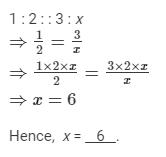Question 22: Fill in the blanks:

Ratio of Rs.2 and 50 paise and Rs.3 and 75 paise is ............

ANSWER: Rs.2 and 50 paise = (2 × 100 + 50) paise

= 250 paise

Rs.3 and 75 paise = (3 × 100 + 75) paise

= 375 paise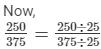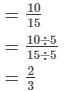Hence. Ratio of Rs.2 and 50 paise and Rs.3 and 75 paise is 2 : 3.

Question 23: Fill in the blanks:
Mean proportion of 2 and 8 is ..........

ANSWER: Let the mean proportion of 2 and 8 be x.
Then,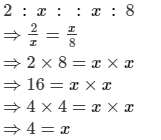Hence, mean proportion of 2 and 8 is 4.

Question 24: If a : b = 4 : 5 and b : c = 2 : 3, then a : c = ...........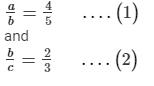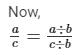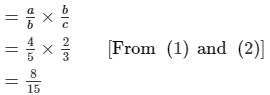Hence, a : c = 8 : 15.

Question 25: Fill in the blanks:

If 2x = 5y, then x : y = ........

2x = 5y         ......(1)
Now,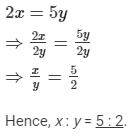Offer running on EduRev: Apply code STAYHOME200 to get INR 200 off on our premium plan EduRev Infinity!

103 docs

,

,

,

,

,

,

,

,

,

,

,

,

,

,

,

,

,

,

,

,

,

,

,

,

,

,

,

,

,

,

;